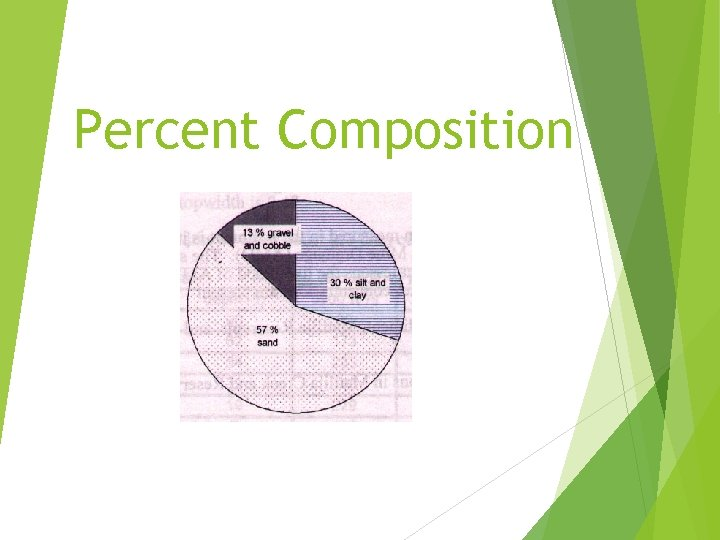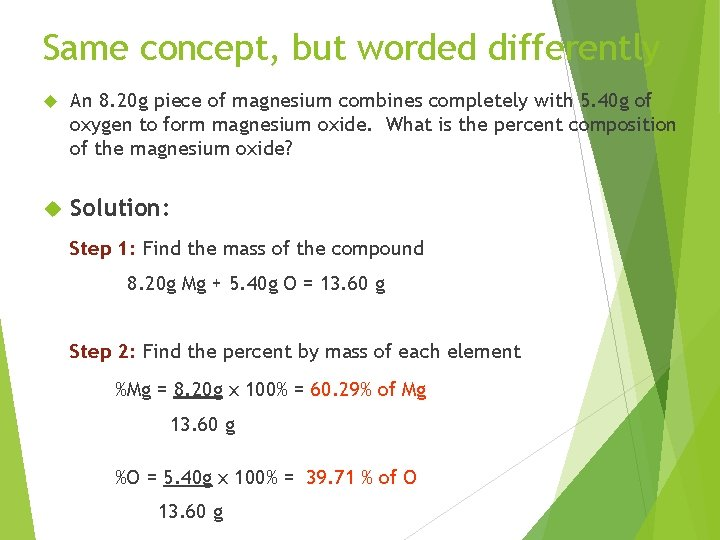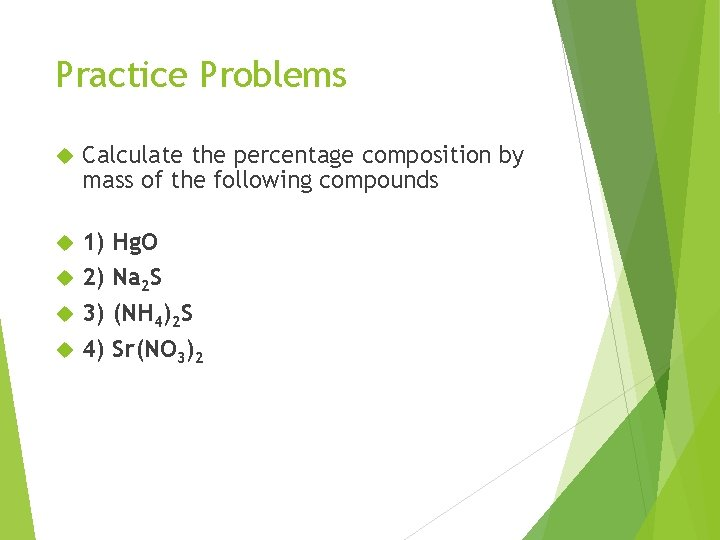# Percent Composition What is Percent Composition The percent

• Slides: 6Percent CompositionWhat is Percent Composition? The percent composition by mass of a compound represents the percent that each element in a compound contributes to the total mass of the compound.How do I calculate percent composition? Formula: %mass = grams of element in 1 mol compound x 100% molar mass of compound Example: What is the percent composition of water (H 2 O)? Step 1: figure out the molar mass from the formula 2 mol Hydrogen x 1. 01 g = 2. 02 g 1 mol Oxygen x 16. 00 g = 16. 00 g molar mass of water = 18. 02 g Step 2: divide the answer for each atom by the molar mass and multiply by 100 to get a percentage 2. 02 g of H / 18. 02 g of H 2 O x 100 % = 11. 21% Hydrogen 16. 00 g of O / 18. 02 g of H 2 O x 100 % = 88. 79% Oxygen Your percentages should add up to 100%Same concept, but worded differently An 8. 20 g piece of magnesium combines completely with 5. 40 g of oxygen to form magnesium oxide. What is the percent composition of the magnesium oxide? Solution: Step 1: Find the mass of the compound 8. 20 g Mg + 5. 40 g O = 13. 60 g Step 2: Find the percent by mass of each element %Mg = 8. 20 g x 100% = 60. 29% of Mg 13. 60 g %O = 5. 40 g x 100% = 39. 71 % of O 13. 60 gYour Turn: What is the percent composition of glucose (C 6 H 12 O 6) Step 1: figure out the molar mass: Step 2: Divide the answer of each atom by the molar mass and multiply by 100Practice Problems Calculate the percentage composition by mass of the following compounds 1) 2) 3) 4) Hg. O Na 2 S (NH 4)2 S Sr(NO 3)2